Before we get back into graphics, it is important to understand some of the fundamentals behind what R is doing.

Please open the `02_graphics.R` script in your working directory. If you cannot find this file, you may need to do some or all of the following:

``````setwd(choose.dir(getwd())) # change your working directory
ISDSWorkshop::workshop()   # write the files (and open up the workshop outline)``````

## Data types

Objects in R can be broadly classified according to their dimensions:

• scalar
• vector
• matrix
• array (higher dimensional matrix)

and according to the type of variable they contain:

• integer
• numeric
• character (string)
• logical
• factor

### Scalars

Scalars have a single value assigned to the object in R.

``````a = 3.14159265
b = "ISDS Workshop"
c = TRUE``````

Print the objects

``a``
``##  3.141593``
``b``
``##  "ISDS Workshop"``
``c``
``##  TRUE``

### Vectors

The `c()` function creates a vector in R

``````a = c(1,2,-5,3.6)
b = c("ISDS","Workshop")
c = c(TRUE, FALSE, TRUE)``````

To determine the length of a vector in R use `length()`

``length(a)``
``##  4``
``length(b)``
``##  2``
``length(c)``
``##  3``

To determine the type of a vector in R use `class()`

``class(a)``
``##  "numeric"``
``class(b)``
``##  "character"``
``class(c)``
``##  "logical"``

#### Vector construction

Create a numeric vector that is a sequence using : or `seq()`.

``1:10``
``##    1  2  3  4  5  6  7  8  9 10``
``5:-2``
``##   5  4  3  2  1  0 -1 -2``
``seq(from = 2, to = 5, by = .05)``
``````##   2.00 2.05 2.10 2.15 2.20 2.25 2.30 2.35 2.40 2.45 2.50 2.55 2.60 2.65
##  2.70 2.75 2.80 2.85 2.90 2.95 3.00 3.05 3.10 3.15 3.20 3.25 3.30 3.35
##  3.40 3.45 3.50 3.55 3.60 3.65 3.70 3.75 3.80 3.85 3.90 3.95 4.00 4.05
##  4.10 4.15 4.20 4.25 4.30 4.35 4.40 4.45 4.50 4.55 4.60 4.65 4.70 4.75
##  4.80 4.85 4.90 4.95 5.00``````

Another useful function to create vectors is `rep()`

``rep(1:4, times = 2)``
``##  1 2 3 4 1 2 3 4``
``rep(1:4, each  = 2)``
``##  1 1 2 2 3 3 4 4``
``rep(1:4, each  = 2, times = 2)``
``##   1 1 2 2 3 3 4 4 1 1 2 2 3 3 4 4``

Arguments to functions in R can be referenced either by position or by name or both. The safest and easiest to read approach is to name all your arguments. I will often name all but the first argument.

#### Accessing vector elements

Elements of a vector can be accessed using brackets, e.g. [index].

``````a = c("one","two","three","four","five")
a``````
``##  "one"``
``a[2:4]``
``##  "two"   "three" "four"``
``a[c(3,5)]``
``##  "three" "five"``
``a[rep(3,4)]``
``##  "three" "three" "three" "three"``

Alternatively we can access elements using a logical vector where only TRUE elements are accessed.

``a[c(TRUE, TRUE, FALSE, FALSE, FALSE)]``
``##  "one" "two"``

You can also remove elements using a negative sign `-`.

``a[-1]``
``##  "two"   "three" "four"  "five"``
``a[-(2:3)]``
``##  "one"  "four" "five"``

#### Modifying elements of a vector

You can assign new values to elements in a vector using = or <-.

``````a = "twenty-two"
a``````
``##  "one"        "twenty-two" "three"      "four"       "five"``
``````a[3:4] = "three-four" # assigns "three-four" to both the 3rd and 4th elements
a``````
``##  "one"        "twenty-two" "three-four" "three-four" "five"``
``````a[c(3,5)] = c("thirty-three","fifty-five")
a``````
``````##  "one"          "twenty-two"   "thirty-three" "three-four"
##  "fifty-five"``````

### Matrices

Matrices can be constructed using `cbind()`, `rbind()`, and `matrix()`:

``````m1 = cbind(c(1,2), c(3,4))       # Column bind
m2 = rbind(c(1,3), c(2,4))       # Row bind

m1``````
``````##      [,1] [,2]
## [1,]    1    3
## [2,]    2    4``````
``all.equal(m1, m2)``
``##  TRUE``
``````m3 = matrix(1:4, nrow = 2, ncol = 2)
all.equal(m1, m3)``````
``##  TRUE``
``````m4 = matrix(1:4, nrow = 2, ncol = 2, byrow = TRUE)
all.equal(m3, m4)``````
``##  "Mean relative difference: 0.4"``
``m3``
``````##      [,1] [,2]
## [1,]    1    3
## [2,]    2    4``````
``m4``
``````##      [,1] [,2]
## [1,]    1    2
## [2,]    3    4``````

#### Accessing matrix elements

Elements of a matrix can be accessed using brackets separated by a comma, e.g. [row index, column index].

``````m = matrix(1:12, nrow=3, ncol=4)
m``````
``````##      [,1] [,2] [,3] [,4]
## [1,]    1    4    7   10
## [2,]    2    5    8   11
## [3,]    3    6    9   12``````
``m[2,3]``
``##  8``

Multiple elements can be accessed at once

``m[1:2,3:4]``
``````##      [,1] [,2]
## [1,]    7   10
## [2,]    8   11``````

If no row (column) index is provided, then the whole row (column) is accessed.

``m[1:2,]``
``````##      [,1] [,2] [,3] [,4]
## [1,]    1    4    7   10
## [2,]    2    5    8   11``````

Like vectors, you can eliminate rows (or columns)

``m[-c(3,4),]``
``````##      [,1] [,2] [,3] [,4]
## [1,]    1    4    7   10
## [2,]    2    5    8   11``````

Be careful not to forget the comma, e.g.

``m[1:4]``
``##  1 2 3 4``

You can also construct an object with more than 2 dimensions using the `array()` function.

### Cannot mix types

You cannot mix types within a vector, matrix, or array

``c(1,"a")``
``##  "1" "a"``

The number 1 is in quotes indicating that R is treating it as a character rather than a numeric.

``c(TRUE, 1, FALSE)``
``##  1 1 0``

The logicals are converted to numeric (0 for FALSE and 1 for TRUE).

``c(TRUE, 1, "a")``
``##  "TRUE" "1"    "a"``

Everything is converted to a character.

### Activity

Reconstruct the following matrix using the `matrix()` function, then

1. Print the element in the 3rd-row and 4th column
2. Print the 2nd column
3. Print all but the 3rd row
``````m = rbind(c(1, 12, 8, 6),
c(4, 10, 2, 9),
c(11, 3, 5, 7))
m``````
``````##      [,1] [,2] [,3] [,4]
## [1,]    1   12    8    6
## [2,]    4   10    2    9
## [3,]   11    3    5    7``````

When you have completed the activity, compare your results to the solutions.

## Data frames

A `data.frame` is a special type of matrix that allows different data types in different columns.

We have already seen a `data.frame` with our GI data set. Let’s read this data in again and take a look.

``````GI = read.csv("GI.csv")
dim(GI)``````
``##  21244     9``

### Access `data.frame` elements

A `data.frame` can be accessed just like a matrix, e.g. [row index, column index].

``GI[1:2, 3:4]``
``````##   facility   icd9
## 1       67 787.01
## 2       67 558.90``````

`data.frame`s can also be accessed by column names

``GI[1:2, c("facility","icd9","gender")]``
``````##   facility   icd9 gender
## 1       67 787.01   Male
## 2       67 558.90 Female``````

or

``````library('dplyr')
GI %>%
select(facility, icd9, gender) %>%
``````##   facility   icd9 gender
## 1       67 787.01   Male
## 2       67 558.90 Female``````

The `%>%` (pipe) operator allows chaining of commands by passing the result of the previous command as the first argument of the next command. This makes code much easier to read. Two equivalent approaches that are harder to read are

``````# Approach 1
head(select(GI, facility, icd9, gender), n = 2)

# Approach 2
GI_select <- select(GI, facility, icd9, gender)
head(GI_select, n = 2)``````

When there are long strings of commands, using the `%>%` (pipe) operator makes code much easier to read. See here for more background and information.

### Different data types in different columns

The function `str()` allows you to see the structure of any object in R. Using `str()` on a `data.frame` object tells you

1. that the object is a `data.frame`,
2. the number of rows and columns,
3. the names of each column,
4. each column’s data type, and
5. the first few elements in that column.
``str(GI)``
``````## 'data.frame':    21244 obs. of  9 variables:
##  \$ id             : int  1001301988 1001829757 1001581758 1001950471 1001076304 1001087075 1001536948 1001966448 1001868408 1001356109 ...
##  \$ date           : Factor w/ 1399 levels "2005-02-28","2005-03-01",..: 1 1 1 1 1 2 2 2 2 2 ...
##  \$ facility       : int  67 67 123 123 309 66 6201 67 66 66 ...
##  \$ icd9           : num  787 559 788 788 559 ...
##  \$ age            : int  7 41 2 71 28 43 12 1 25 64 ...
##  \$ zipcode        : int  21075 20721 22152 22060 21702 20762 22192 20121 20772 20602 ...
##  \$ chief_complaint: Factor w/ 2936 levels " /V/D"," /v",..: 784 2904 2759 9 1646 2229 601 2876 1736 2166 ...
##  \$ syndrome       : Factor w/ 1 level "GI": 1 1 1 1 1 1 1 1 1 1 ...
##  \$ gender         : Factor w/ 2 levels "Female","Male": 2 1 2 2 1 1 2 2 2 2 ...``````

### Factor

A factor is a data type that represents a categorical variable.

The default is for any character vector to be converted to a factor when read using `read.csv()` or `read.table()`. You can change this behavior by setting `stringsAsFactors = FALSE` and this is the default in `readr::read_csv()`.

Internally, R codes a factor as an integer and then keeps a table that contains the conversion from that integer into the actual value of the factor.

``nlevels(GI\$gender)``
``##  2``
``levels(GI\$gender)          # internal table``
``##  "Female" "Male"``
``GI\$gender[1:3]``
``````##  Male   Female Male
## Levels: Female Male``````
``as.numeric(GI\$gender[1:3]) # internal coding``
``##  2 1 2``

#### Converting a numeric variable into a factor

When a categorical variable is encoded as a numeric variable in the original data set, R reads them in as numeric. To convert them to a factor use `as.factor()` or `factor()`.

``````GI\$facility = as.factor(GI\$facility)
summary(GI\$facility)``````
``````##   37   66   67  123  255  256  259  309  390  413  420  522  703 6200 6201
## 3571 2950 4281 4408   41  575    1  325  661  668  100   67  178 1423 1928
## 7298
##   67``````

#### Converting back to the original numeric variable

To obtain the original numeric variable use `as.character()` and `as.numeric()`

``head(as.character(GI\$facility))             # This returns the levels as a character vector``
``##  "67"  "67"  "123" "123" "309" "66"``
``head(as.numeric(as.character(GI\$facility))) # This returns the original numeric factor levels``
``##   67  67 123 123 309  66``

#### Creating your own factor

Use the `cut()` function to create a factor from a continuous variable.

``````GI\$ageC = cut(GI\$age, c(-Inf, 5, 18, 45 ,60, Inf)) # Inf is infinity
table(GI\$ageC)``````
``````##
##  (-Inf,5]    (5,18]   (18,45]   (45,60] (60, Inf]
##      4324      3802      7476      3133      2509``````

This created a new variable in the GI `data.frame` called ageC.

### Dates

In order to use dates properly, they need to be converted into type `Date`.

``````GI\$date = as.Date(GI\$date)
str(GI\$date)``````
``##  Date[1:21244], format: "2005-02-28" "2005-02-28" "2005-02-28" "2005-02-28" ...``

`as.Date()` will attempt to read dates as “%Y-%m-%d” then “%Y/%m/%d”. If neither works, it will give an error.

``?as.Date``

You can specify other date patterns, e.g.

``as.Date("12/09/14", format="%m/%d/%y")``

For those who work with dates often, check out the lubridate package. To convert from dates to MMWR weeks, check out the MMWRweek package.

### Activity

Create a new variable in the GI data set called `icd9code` that cuts icd9 at 0, 140, 240, 280, 290, 320, 360, 390, 460, 520, 580, 630, 680, 710, 740, 760, 780, 800, 1000, and Inf. Find the `icd9code` that is the most numerous in the GI data set.

``````# Create icd9code

# Find the icd9code that is most numerous``````

When you have completed the activity, compare your results to the solutions.

## Reshaping data frames in R

There are two general representations of tabular data.

Wide:

``````##   week  GI  ILI
## 1    1 246  948
## 2    2 195 1020
## 3    3 212 1024``````

which is a succinct representation of the data

Long:

``````##
## Attaching package: 'tidyr'``````
``````## The following object is masked _by_ '.GlobalEnv':
##
##     population``````
``````##   week syndrome count
## 1    1       GI   246
## 2    2       GI   195
## 3    3       GI   212
## 4    1      ILI   948
## 5    2      ILI  1020
## 6    3      ILI  1024``````

which is the form most statistical software wants, i.e. there is only one column for the response (count).

### Wide to long

The `tidyr` package provides functions to convert between the two representations. First, we need to load the package

``library('tidyr')``

Create the wide `data.frame`:

``````d = data.frame(week = 1:3,
GI   = c(246,195,212),
ILI  = c(948, 1020, 1024))``````

To turn the `data.frame` into long format, use `gather()`.

``````m <- d %>%
gather(key = syndrome, # Creates a column called syndrome
value = count,  # Creates a column called count
-week)          # Keeps the column `week` as a column
# All other columns (GI and ILI) are gathered``````

This approach is useful if there are a lot of columns that need to be gathered but only a few that need to remain as columns. If the opposite is true, i.e. there are only a few columns to be gathered and a lot that need to remain as columns, use

``````m2 <- d %>%
gather(key = syndrome, # Creates a column called syndrome
value = count,  # Creates a column called count
GI, ILI)        # Gathers these columns

all.equal(m,m2)``````
``##  TRUE``

### Long to wide

If we want to convert back, use `spread()`

``````m %>%
spread(key = syndrome, value = count)``````
``````##   week  GI  ILI
## 1    1 246  948
## 2    2 195 1020
## 3    3 212 1024``````

I find that I use the `gather` function much more often than I use the `spread` function because data are usually stored in a succinct format but then I need the data in long format for summaries or figures or statistical analyses.

## Aggregating data frames in R

The GI data set that we have is already in long format and each row is an individual. We may want to aggregate this information. To do so, we will use the `group_by()` and `summarize()` functions in the `dplyr` package.

``library('dplyr')``

For example, perhaps we wanted to know the total number of GI or ILI cases across the 3 weeks:

``````m %>%                           # We need to use the melted (long) version of the data set
group_by(syndrome) %>%        # Do the following for each syndrome
summarize(total = sum(count)) # Calculate `total` which is the sum of count for each syndrome``````
``````## # A tibble: 2 × 2
##   syndrome total
##      <chr> <dbl>
## 1       GI   653
## 2      ILI  2992``````

### Aggregating the GI data set

Let’s aggregate the GI data set by week, gender, and age category.

First, we need to create weeks

``````GI\$date = as.Date(GI\$date) # Make sure the dates are actually dates
GI\$week = cut(GI\$date,
breaks = "weeks",
start.on.monday = TRUE) ``````

Now we can summarize

``````GI_count <- GI %>%                 # each row is a single observation
group_by(week, gender, ageC) %>% # split the data by these variables
summarize(total = n())           # this counts the number of rows, see ?n

nrow(GI_count)``````
``##  2008``
``head(GI_count, 20)``
``````## Source: local data frame [20 x 4]
## Groups: week, gender 
##
##          week gender      ageC total
##        <fctr> <fctr>    <fctr> <int>
## 1  2005-02-28 Female  (-Inf,5]     9
## 2  2005-02-28 Female    (5,18]     7
## 3  2005-02-28 Female   (18,45]    20
## 4  2005-02-28 Female   (45,60]     5
## 5  2005-02-28 Female (60, Inf]     5
## 6  2005-02-28   Male  (-Inf,5]    11
## 7  2005-02-28   Male    (5,18]     9
## 8  2005-02-28   Male   (18,45]    12
## 9  2005-02-28   Male   (45,60]     3
## 10 2005-02-28   Male (60, Inf]     5
## 11 2005-03-07 Female  (-Inf,5]     9
## 12 2005-03-07 Female    (5,18]     9
## 13 2005-03-07 Female   (18,45]    29
## 14 2005-03-07 Female   (45,60]    15
## 15 2005-03-07 Female (60, Inf]     8
## 16 2005-03-07   Male  (-Inf,5]    18
## 17 2005-03-07   Male    (5,18]    12
## 18 2005-03-07   Male   (18,45]    18
## 19 2005-03-07   Male   (45,60]     9
## 20 2005-03-07   Male (60, Inf]     6``````

### Activity

Aggregate the GI data set by gender, ageC, and icd9code (the ones created in the last activity).

When you have completed the activity, compare your results to the solutions.

## Basics of `ggplot2`

Previously we produced graphics using the base `graphics` system. Although I still use this for producing quick plots, I invariably end up using the `ggplot2` package. This package requires a `data.frame` in long format.

Load the `ggplot2` package

``library('ggplot2')``

### Histogram

A basic histogram in ggplot

``ggplot(data = GI, aes(x = age)) + geom_histogram(binwidth = 1)``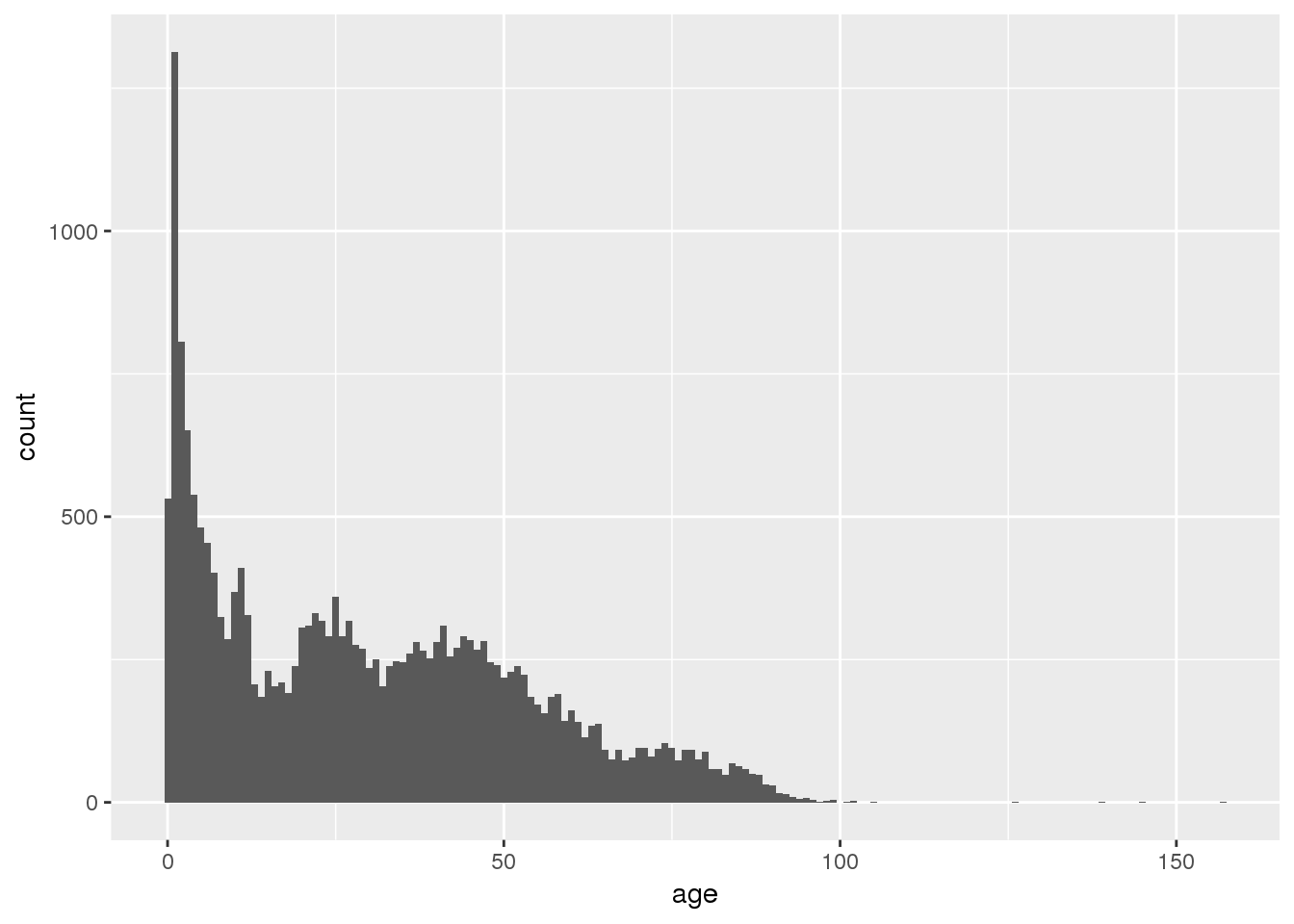For code that looks more similar to the histogram code we saw before, you can use

``qplot(data = GI, x = age, geom = "histogram", binwidth = 1)``

Many websites and even the `ggplot2` manual have examples using `qplot`. I believe this is mainly to ease the transition for individuals who are familiar with base `graphics`. If you are just starting out with R, I recommend using the `ggplot` function in `ggplot2` from the beginning.

### Boxplots

A basic boxplot

``ggplot(data = GI, aes(x = 1, y = age)) + geom_boxplot()``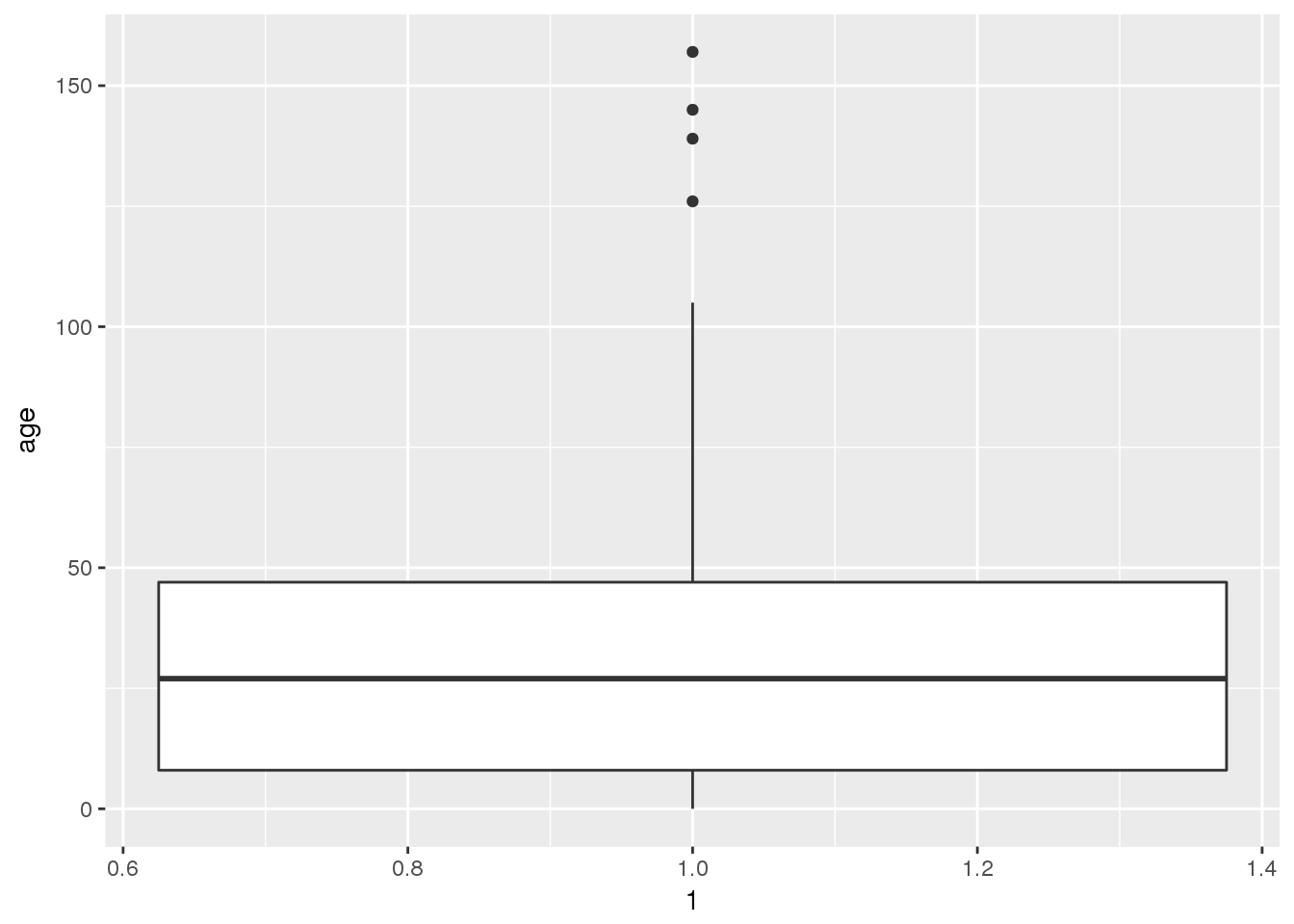### Multiple boxplots

``ggplot(GI, aes(x = facility, y = age)) + geom_boxplot()``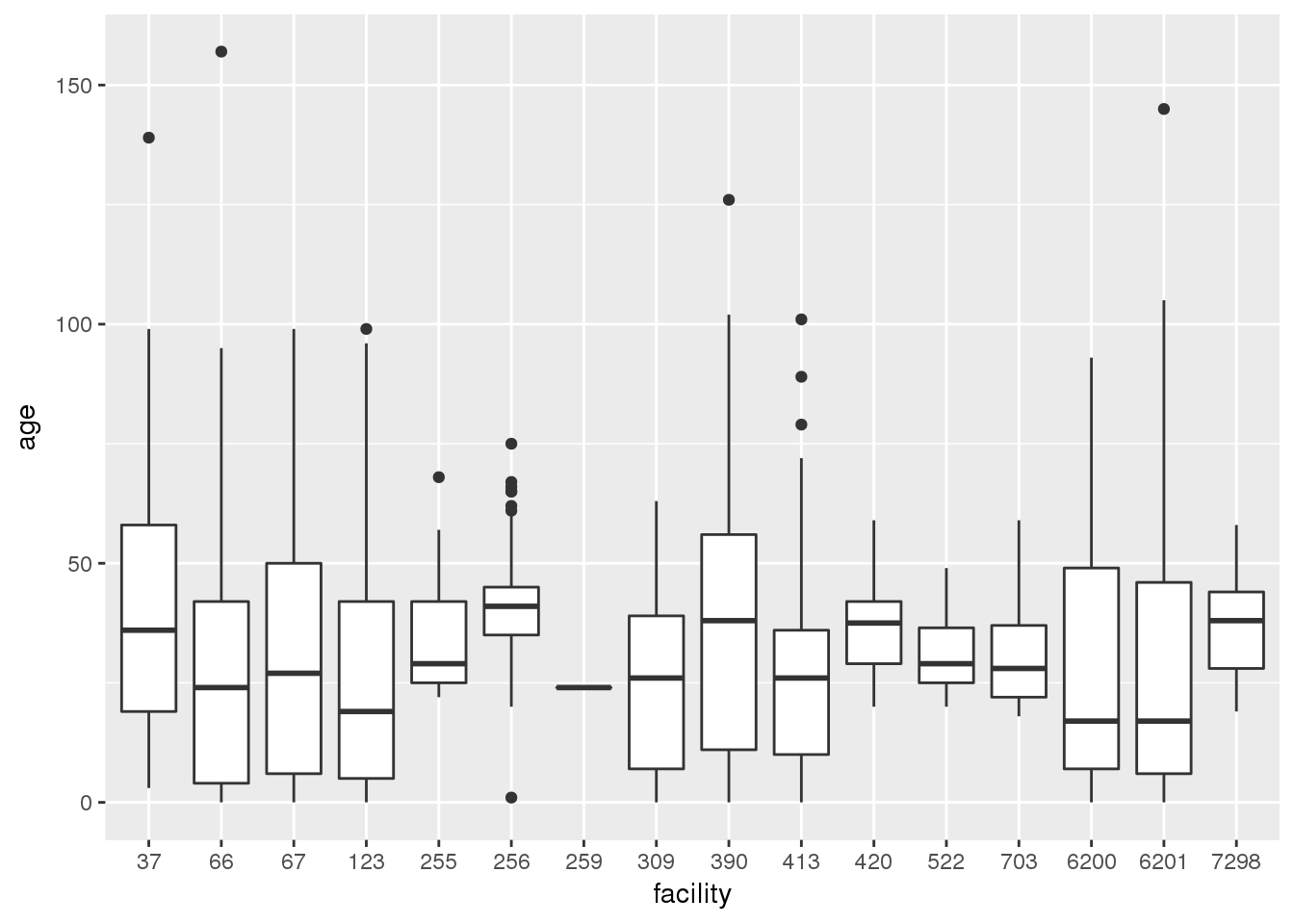### Scatterplots

``ggplot(GI, aes(x=date, y=age)) + geom_point()``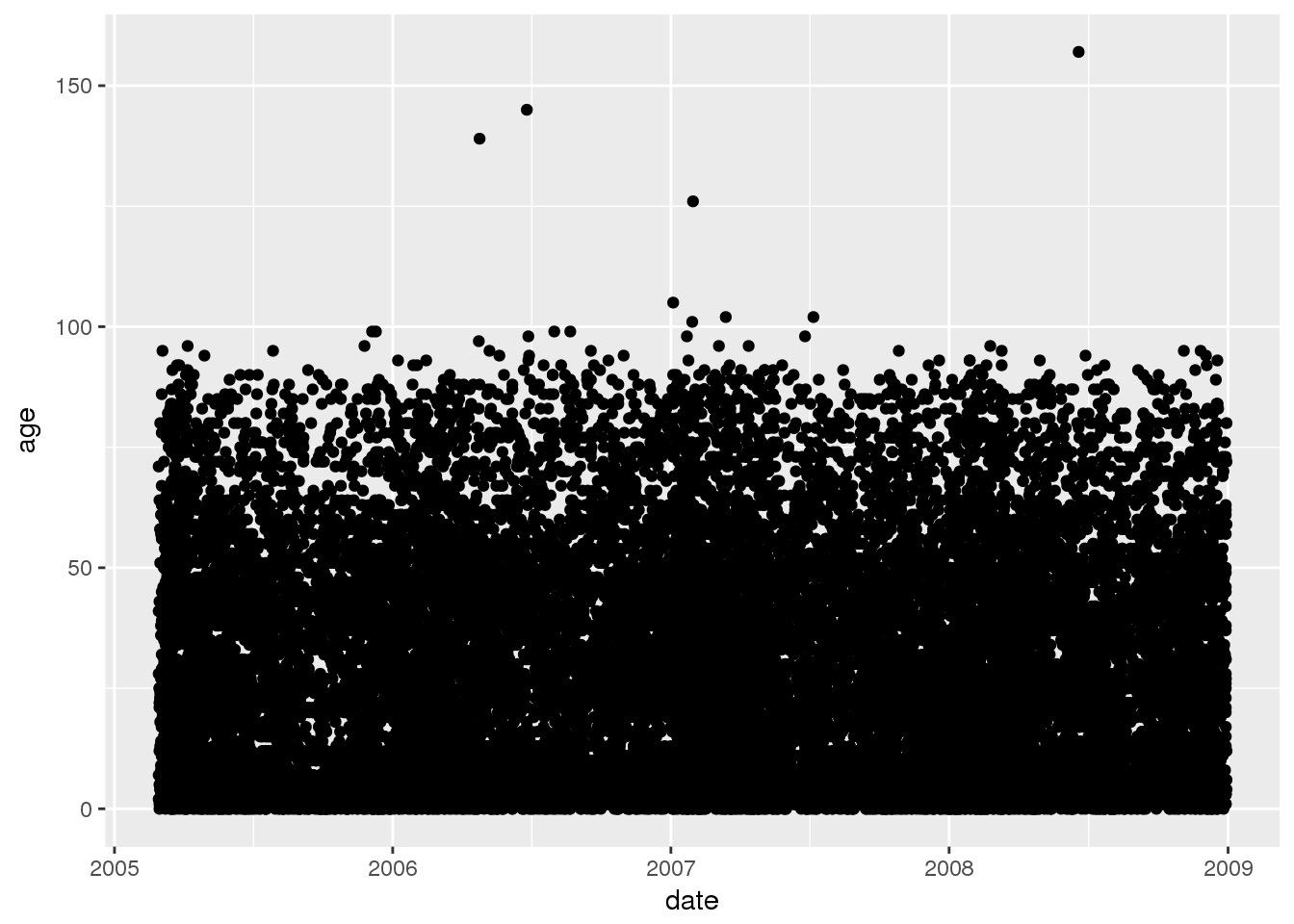### Bar charts

With ggplot, there is no need to count first.

``ggplot(GI, aes(x=facility)) + geom_bar()``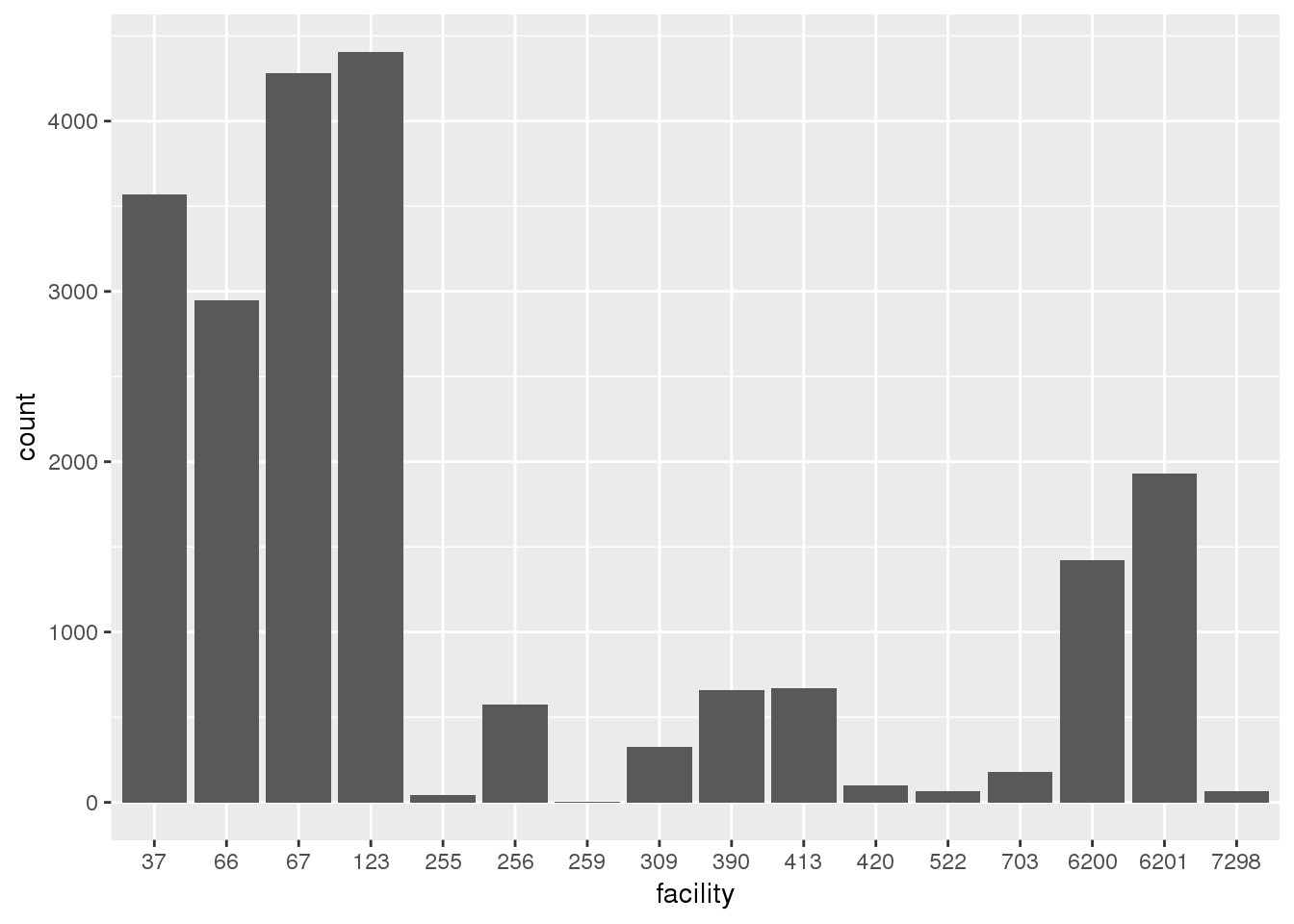An appealing aspect of ``ggplot` is that once the data is in the correct format it is easy to construct lots of different plots.

### Activity

Construct a histogram and boxplot for age at facility 37 using ggplot2.

``````# Construct a histogram for age at facility 37.

# Construct a boxplot for age at facility 37. ``````

Construct a bar chart for the 3-digit zipcode at facility 37 using ggplot2

``# Construct a bar chart for the 3-digit zipcode at facility 37 ``

When you have completed the activity, compare your results to the solutions.

## Customizing ggplot2 plots

There are many ways to customize the appearance of ggplot2 plots:

• Colors
• Labels
• Titles
• Characters
• Line types
• Themes

### Colors

``````ggplot(GI, aes(x = age)) +
geom_histogram(binwidth = 1, color = 'blue',   fill = 'yellow')``````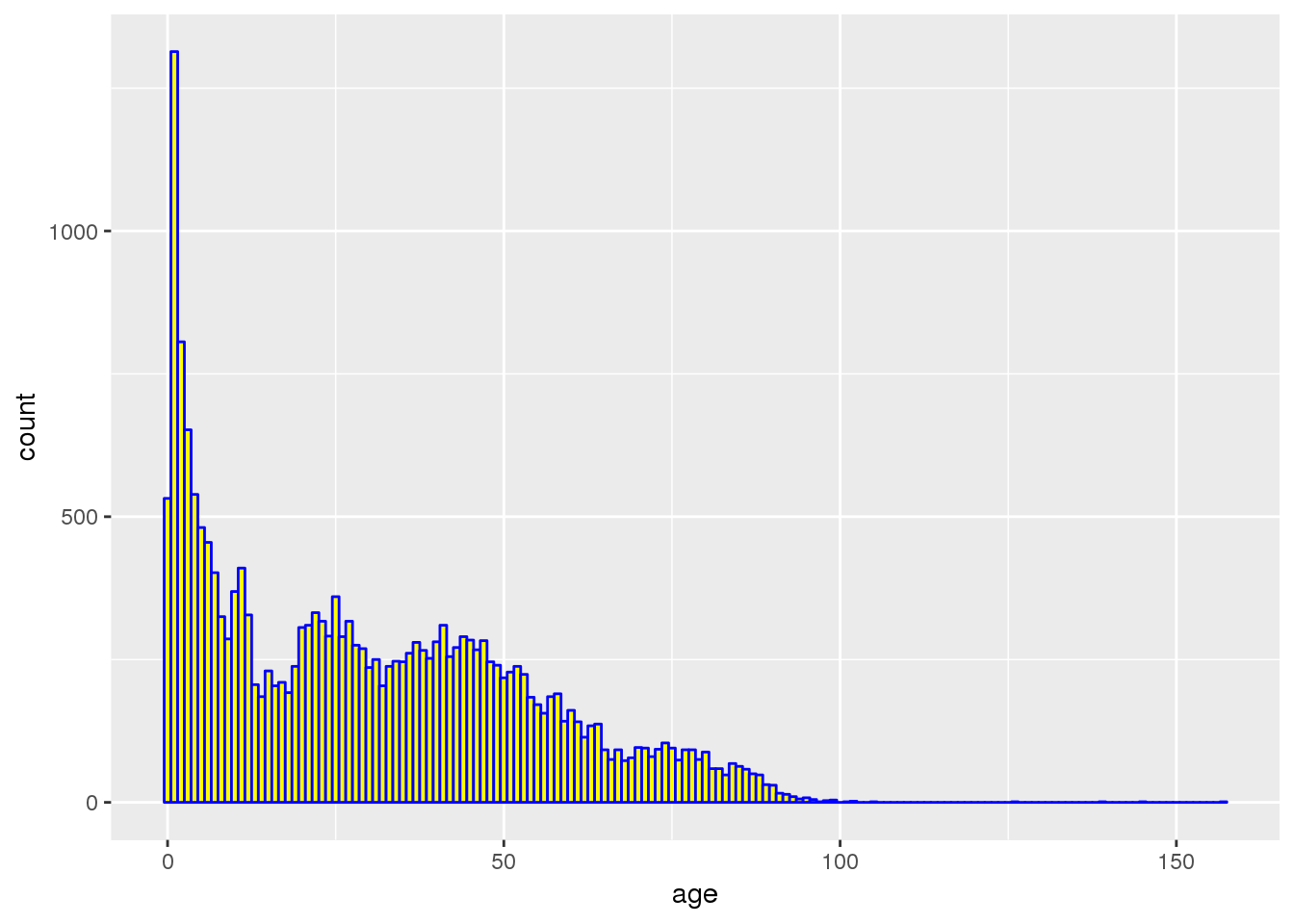``````ggplot(GI, aes(x=date, y=age)) +
geom_point(color = 'purple')``````